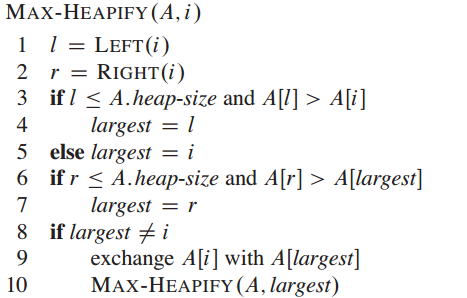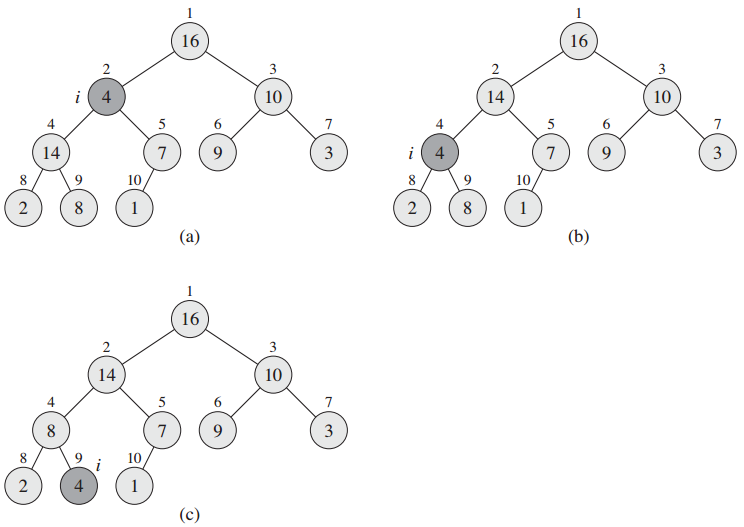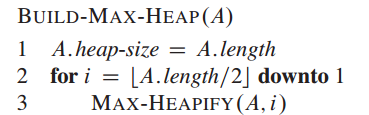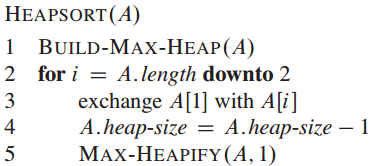## 堆(6.1, P84)## 维持堆的性质（6.2，P86）

MAX-HEAPIFY：输入为一个数组A和一个下标i，A[i]有可能小于其孩子，通过让A[i]在数组中“逐级下降”，从而使得以下标i为根节点的子树重新遵循最大堆的性质。Python实现为：

$$T(n) = T(2n/3)+ \Theta(1)$$

## 建堆(6.3, P87)

BUILD_MAX_HEAP从非叶节点开始一直循环到根节点。Python实现为：

BUILD_MAX_HEAP 的时间复杂度为$T(n) = \text{O}(n)$

## 堆排序算法(6.4，P89)Python实现：

HEAPSORT过程的时间复杂度为：$\text{O}(n\text{lg}n)$，因为BUILD_MAX_HEAP的时间复杂度为$\text{O}(n)$，n-1次调用MAX_HEAPIFY，每次时间为$\text{O}(\text{lg}n)$。

## 优先队列(6.5，P90)

• 对最大优先队列进行插入，MaxHeapInsert
• 返回最大优先队列的最大值，HeapMax
• 去掉最大值并且返回该值，HeapExtractMax
• 将第x个元素的值改为k，其中k>=x的原来的值，HeapIncreaseKey

HeapExtractMax的操作复杂度为$\text{O}(\text{lg}n)$（也就是MAX_HEAPIFY的复杂度）。

## 参考资料

------ 本文结束感谢您的阅读 ------